# AP Physics 1 Question 316: Answer and Explanation

### Test Information

Question: 316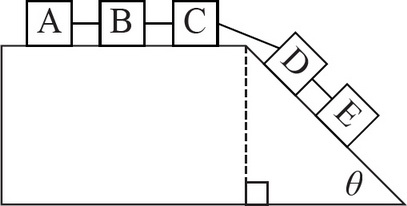4. Five boxes are linked together, as shown above. If both the flat and slanted portions of the surface are frictionless, what will be the acceleration of the box marked B ?

• A.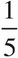g sinθ
• B.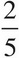g sinθ
• C.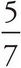g sinθ
• D.g cosθ

The normal force and the perpendicular component of gravity will cancel out. This leaves just the parallel component of gravity to be a net force. Using Newton's Second Law, you get FNet = Fg,parallel 2mg sin θ = 5ma. The 2 comes in because there are two blocks on the ramp and each one will contribute the net force discussed earlier. The 5 is necessary because the blocks cannot accelerate individually. Either they all move or none do. Solving for a then gives a =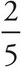gsinθ.